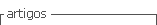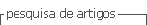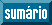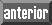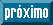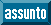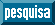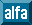## Artigo

•Citado por SciELO
•Acessos

•Citado por Google
•Similares em SciELO
•Similares em Google

## versão impressa ISSN 0120-419X

### Integración - UIS vol.35 no.2 Bucaramanga jul./dez. 2017

#### http://dx.doi.org/10.18273/revint.v35n2-2017001

Artículos originales

When is R[x] a principal ideal ring?

¿Cuándo R[x] es un anillo de ideales principales?

Henry Chimal-Dzul1  , C. A. López-Andrade2

1Ohio University, Department of Mathematics, Athens, USA

2Benemérita Universidad Autónoma de Puebla, Facultad de Ciencias Físico Matemáticas, Puebla, México

Abstract

Because of its interesting applications in coding theory, cryptography, and algebraic combinatorics, in recent decades a lot of attention has been paid to the algebraic structure of the ring of polynomials R[x], where R is a finite commutative ring with identity. Motivated by this popularity, in this paper we determine when R[x] is a principal ideal ring. In fact, we prove that R[x] is a principal ideal ring if and only if R is a finite direct product of finite fields.

MSC2010: 13F10, 13F20, 16P10, 13C05.

Key words: Principal ideal ring; polynomial ring; finite rings

Resumen

Debido a sus interesantes aplicaciones en teoría de códigos, criptografía y combinatoria algebraica, en décadas recientes se ha incrementado la atención en la estructura algebraica del anillo de polinomios R[x], donde R es un anillo conmutativo finito con identidad. Motivados por esta popularidad, en este artículo determinamos cuándo R[x] es un anillo de ideales principales. De hecho, demostramos que R[x] es un anillo de ideales principales, si y sólo si, R es un producto directo finito de campos finitos.

Palabras-clave: Anillo de ideales principales; anillo de polinomios; anillos finitos

1. Introduction and preliminaries

Polynomials with coefficients in a finite commutative ring R with identity arise naturally, for instance, in practical applications dealing with coding theory, cryptography and algebraic combinatorics, e. g. , , , , . For some of these applications, it is important to know the algebraic structure of either the ring of polynomials R[x] or the quotient ring R[x]/A, where A is an ideal of R[x]. In particular, often one wants to know when such rings are principal ideal rings (PIR’s). Motivated by this question, in this paper we examine when R[x] is a PIR. We prove that R[x] is a principal ideal ring if and only if R is a finite direct product of finite fields. To this end, let us start remembering some facts about commutative finite rings with identity.

The most familiar example of a finite commutative ring with identity is the ring Z m of integers modulo m ≥ 2. When m is a composite number, the Chinese Remainder Theorem assures that

where m = p 1 α1 · · · pk αk is the prime factorization of m, and Z p1 α1 ×· · ·× Zpk αk is the direct product of the rings Zpi αi , 1 ≤ in. Remember that for each i (1 ≤ i ≤ k), Zpi αi is a finite local ring with maximal ideal(see ). In particular, note that if α = 1, then Zp is a finite field (see ). Consequently, the Chinese Remainder Theorem establishes that Zm is isomorphic to a direct product of finite local rings. This is the simplest case of the following result.

Theorem 1.1 (Structure of Finite Commutative Rings, , Theorem VI.2). Every finite commutative ring with identity is isomorphic to a direct product of finite commutative local rings with identity. This decomposition is unique up to the order of the factors.

In view of the previous theorem, if R is a finite commutative ring with identity and R 1 × · · · × Rn is the unique decomposition of R as a direct product of local rings, then there exists a ring isomorphism φ: RR 1 × · · · × Rn which maps each element rR into a unique n-tuple φ(r) = (r 1, . . . , rn ) ∈ R 1 × · · · × Rn . This map extends to a ring isomorphism

defined by

where p(x) = p 0 + p 1 x + · · · + pkxk and φ(pi ) = (p 1 (i) , . . . , pn (i)), 0 ≤ ik.

Proposition 1.2. Let R1, . . . ,Rn be commutative rings with identity. Then their direct product R = R1 × · · · × Rn is a PIR if and only if Ri is a PIR for each i, 1 ≤ in.

Proof. Let A be an ideal of R and for each i, 1 ≤ in, define Ai = {ai Ri : (a 1, . . . , ai , . . . , an ) ∈ A}. Then Ai is an ideal of Ri and we claim that A = A 1×· · ·×An . The inclusion AA 1 × · · · × An is clear. To prove the reverse inclusion, let a = (a 1, . . . , an ) be an element in A 1 × · · · × An . It follows from the definition of Ai that there exist α 1, . . . , αn A such that a = α 1 e 1+· · ·+αnen , where ei denotes the element of R with a 1 in the ith coordinate and 0’s elsewhere. Hence, using that A is an ideal of R 1×· · ·×Rn , we have that aA. Therefore A = A 1×· · ·×An , and so A =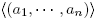if and only if Ai =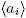for every i, 1 ≤ in.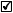In light of Proposition 1.2 and the isomorphism Φ given in (1), R[x] is a PIR if and only if Ri [x] is a PIR for each i, 1 ≤ in. Hence, in order to determine whenever R[x] is a PIR, it is natural to ask when Ri [x] is a PIR. This is the purpose of the next section. To illustrate the results of Section 2, we present in Section 3 three families of finite rings with identity that are relevant in the theory of finite commutative rings.

2. Ideals of R[x]

First of all, by a ring R we will always mean a commutative ring with identity 1 ≠ 0, and remember that a ring R is called local if it has a unique maximal ideal, or equivalently, R is a local ring with maximal ideal M if and only if M = R\U(R), where U(R) denotes the group of units of R. We usually write (R,M) or (R,M, F) to denote a local ring R, its maximal ideal M and its residue field F = R/M (using that M is a maximal ideal of R, the quotient ring R/M is indeed a field).

The simplest case of a finite local ring R is when R is a finite field. In this case the ring R[x] of polynomials with coefficients in R is a PIR. In fact, R[x] is a principal ideal domain (PID). Therefore, in what follows we focus our attention on a finite local ring R which is not a field.

Let (R,M, F) be a finite local ring which is not a field. Note that the natural surjective homomorphism − : RF induces a surjective polynomial ring homomorphism μ: R[x] → F[x] given by

This ring homomorphism lets us deduce some facts about polynomials f(x) = Σ n i =0 fixi ∈ R[x] by using the structure of F[x]. In particular, note that μ(f(x)) = 0 if and only if fi M for all i, 0 ≤ in and so, it follows that ker μ is a proper ideal of R[x] (indeed, ker μ is a prime ideal of R[x] because R[x]/ ker μ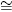F[x] is an integral domain).

On the other hand, remember that an element r of a ring R is called irreducible if it is neither 0 nor a unit in R and the condition r = ab, for some a, bR, implies that aU(R) or bU(R).

Having remembered the above, if f(x) ∈ R[x] is such that μ(f(x)) is irreducible in F[x], then f(x) is also irreducible in R[x]. Since for every finite field K and every positive integer n there exists an irreducible polynomial in K[x] of degree n (see ), we conclude that irreducible polynomials in R[x] of degree n exist for every positive integer n. It is worth to mention that the converse of this fact does not hold in general. That is, if f(x) is an irreducible polynomial in R[x] then μ(f(x)) is not necessarily irreducible in F[x]. For instance, if p ≥ 2 is a prime number, then p ∈ Z p² [x] is irreducible in Zp² [x] but μ(p) = 0̅ ∈ (Z p²/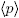[x] is not irreducible by definition. Furthermore, if f(x) = x 2 + 2x + 3 ∈ Z4[x] then f(x) is irreducible in Z4[x]; whereas μ(f(x)) = x 2 + 1̅ = (x + 1̅)2 ∈ (Z4/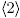) [x] is not irreducible.

Lemma 2.1. Let (R,M) be a local ring which is not a field, p(x) an irreducible polynomial in R[x] and θM \ {0}. Then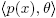is a proper ideal of R[x].

Proof. If μ(p(x)) = 0̅, then p(x) ∈ ker μ, so that⊆ ker μ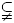R[x]. If μ(p(x)) ≠ 0̅, then we proceed by contradiction. Assume then the existence of some polynomials f(x), g(x) ∈ R[x] such that 1 = f(x)θ + g(x)p(x). This implies that 1̅ = μ(g(x))μ(p(x)), which contradicts the irreducibility of p(x). Hence, for every irreducible polynomial p(x) in R[x] and θ ∈ M \ {0},is a proper ideal of R[x].If p(x) = p 0 + p 1 x + · · · + pnxn R[x] is an irreducible polynomial in R[x] such that μ(p(x)) ≠ 0̅, then deg(μ(p(x))) = k ≥ 1, since μ(p(x)) can not be a unit in F[x]. This implies that pk U(R) and pk +1, . . . , pn M and so, for each nonzero polynomial a(x) ∈ R[x] we have that deg(p(x)a(x)) ≥ k ≥ 1. Consequently, every nonzero polynomial in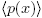R[x] is not a constant polynomial, and so M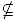.

Theorem 2.2. Let (R,M) be a local ring which is not a field. Then R[x] is not a PIR.

Proof. Let p(x) ∈ R[x] be an irreducible polynomial in R[x] such that μ(p(x)) ≠ 0̅, and let θM \ {0}. Then we claim thatis not a principal ideal of R[x]. Assume the contrary; that is, suppose that there is a polynomial h(x) ∈ R[x] such that=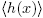. Then there exists f(x) ∈ R[x] such that p(x) = f(x)h(x). Using that p(x) is irreducible, we have that f(x) ∈ U(R[x]) or h(x) ∈ U(R[x]). It follows immediately from Lemma 2.1 that h(x)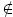U(R[x]), and so f(x) ∈ U(R[x]). This implies that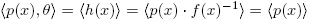, which is a contradiction since θ. In consequence,is not a principal ideal in R[x].Note that we have proved implicitly in Theorem 2.2 that for every local ring (R,M, F) which is not a field, the family of ideals, where p(x) is an irreducible element in R[x] such that μ(p(x)) ≠ 0̅, and θ is a nonzero element in M, consists entirely of non-principal ideals of R[x]. This family contains an infinite number of elements since irreducible polynomials of degree n in R[x] exist for every integer n ≥ 1.

Theorem 2.3. Let R be a finite ring. The following statements are equivalent:

1. R[x] is a PIR.

2. R is isomorphic to a direct product of finite fields.

Proof. It follows immediately from Proposition 1.2 and Theorems 1.1, 2.2.An equivalent way to state Theorem 2.3 is as follows: R[x] is a not a PIR if and only if R is isomorphic to a direct product R 1×· · ·×Ri ×· · ·×Rn of finite local rings such that at least one of them is not a finite field, say (Ri ,Mi , Fi ). In this case, for all θMi \ {0} and for all irreducible polynomials p(x) ∈ Ri [x] with μi (p(x)) ≠ 0̅ in Fi [x] we have thatRi [x] is not a principal ideal in Ri [x]. In consequence, by using the ring isomorphism Φ defined in (1), one can easily show that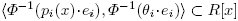is not a principal ideal in R[x].

Another equivalent way to state Theorem 2.3 is derived from the structure theorem for commutative PIR’s due to Zariski-Samuel (see [7, Theorem 33]). This result states that every commutative PIR is (isomorphic to) a direct sum of PID’s and of special PIR’s. Therefore, Theorem 2.3 shows that Zariski-Samuel theorem can be expressed for the ring of polynomials over a finite ring R as follows: R[x] is a PIR if and only if R[x] is isomorphic to a direct product of PID’s. Moreover, the isomorphism Φ given in (1) presents one decomposition of R[x] as a direct product of PID’s. In addition, Theorem 2.2 also implies that for a local ring (R,M) which is not a field, the ring of polynomials R[x] cannot be a special PIR.

3. Examples

As a first example we present the one that we used to motivate Theorem 1.1. Let m ≥ 2 be an integer. Then

where m = p 1 α1 · · · pk αk is the prime factorization of m. Since Z piαi is a finite field if and only if αi = 1, it follows from Theorem 2.3 that Z m [x] is a PIR if and only if α 1 = · · · = αk = 1.

In order to generalize the previous example, let us to consider the ring Z pᵅ , where p is a prime number and α is a positive integer. Let f(u) be a monic polynomial of degree r ≥ 1 in Z pᵅ [u] such that μ(f(u)) is irreducible in (Z p/) [u]. Then the Galois ring of characteristic pα and cardinality pαr is definied as the quotient ring

This ring is a finite local ring with maximal ideal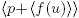, and residue field isomorphic to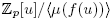(see  for more details). Note that if r = 1 then

Consequently, GR(p, 1)Z p is a finite field, and for any integer α ≥ 2, GR(pα , 1) is a finite local ring which is not a field. Furtheremore, if r ≥ 2 and α = 1, then GR(p, r) = Z p [u]/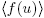is a finite field with pr elements (see ). Hereof it follows from Theorem 2.2 that

Let p 1, p 2, . . . , pk be prime numbers, αi , ri positive integers for 1 ≤ ik, and consider the following ring which is a natural generalization of Z m :

In the examples given above we have presented infinite families of finite rings for which the ring of polynomials with coefficients in these rings are not PIR. The common ground in both families of rings is that they are finite products of local rings whose ideals are principal and they are linearly ordered by inclusion. Any local ring satisfying these conditions is called a finite chain ring (see ). In general, if R is a finite chain ring that is not a field, then R[x] is not a PIR by Theorem 2.2, and so for every finite ring R such that it is isomorphic to a direct product of finite chain rings (of which at least one is not a field), the ring R[x] is not a PIR by Theorem 2.3.

In the following lines, we are going to present a family of finite local rings which was introduced in .

Let p be a prime number, α a positive integer and denote by Fq the unique finite field with q = pα elements (see ). Then for every integer k ≥ 1, the quotient ring Rk = Fq [u 1, u 2, . . . , uk ]/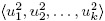is a commutative ring with identity. Furthermore, it is proved in  that Rk is a finite local ring with maximal ideal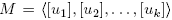and residue field Rk/MF 2. In consequence, Theorem 2.3 shows that Rk [x] is not a PIR for any integer k ≥ 1.

Since k ≥ 2, the ring Rk described above is neither a PIR nor a chain ring in view of M is not a principal ideal and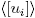and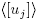are not linearly ordered by inclusion for ij. However, it was pointed out in  that Rk is a finite Frobenius ring.

Acknowledgements

We would like to thank the anonymous referee for his/her useful comments and suggestions that significantly contributed to improve the quality of our manuscript.

References

 Dinh H.Q. and López-Permouth S.R., “Cyclic and negacyclic codes over finite chain rings”, IEEE Trans. Inform. Theory 50 (2004), No. 8, 1728–1744. [ Links ]

 Dougherty S.T., Yildiz B. and Karadeniz S., “Codes over Rk, Gray maps and their binary images”, Finite Fields Appl. 17 (2011), No. 3, 205–219. [ Links ]

 Gómez-Calderón J. and Mullen G. L., “Galois rings and algebraic cryptography”, Acta Arith. 59 (1991), No. 4, 317–328. [ Links ]

 Lidl R. and Niederreiter H., Finite Fields, Cambridge University Press, Cambridge, 1997. [ Links ]

 McDonald B., Finite rings with identity, Marcel Dekker, New York, 1974. [ Links ]

 Xiang-dong H.A. and Nechaev A.A., “A construction of finite Frobenius Rings and its application to partial difference sets”, J. Algebra 309 (2007), No. 1, 1–9. [ Links ]

 Zariski O. and Samuel P., Commutative Algebra I, Springer-Verlag, New York, 1975. [ Links ]

Received: May 05, 2017; Accepted: September 04, 2017This work is under License Creative Commons Attribution 4.0 International.